# 2.2. SUPERSCALAR PROCESSOR

Parallel Computing on Heterogeneous Networks, by Alexey Lastovetsky
ISBN 0-471-22982-2 Copyright 2003 by John Wiley & Sons, Inc.

## 2.1. VECTOR PROCESSOR

The vector processor provides a single control flow with serially executed instructions operating on both vector and scalar operands. The parallelism of this architecture is at the instruction level. Figure 2.1 depicts the architecture. Like the serial scalar processor the vector processor has only one IEU, and this IEU does not begin executing the next instruction until the execution of the current one is completed. Unlike the serial scalar processor, its instructions can operate both on scalar operands and on vector operands. The vector operand is an ordered set of scalars that is generally located on a vector register.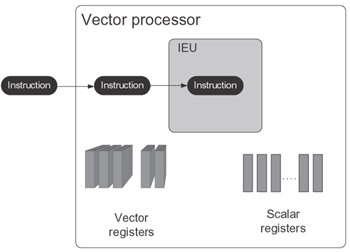Figure 2.1: Vector processor architecture.

While a number of different implementations of this architecture exist, such as ILLIAC-IV, STAR-100, Cyber-205, Fujitsu VP 200, and ATC, probably the most elegant is the vector computer Cray-1 designed by Seymour Cray in 1976. Its processor employs a data pipeline to execute the vector instructions.

The idea of this approach is that the instruction to be executed performs some binary vector operation, say, multiplication, taking operands from vector registers a and b and putting the result on vector register c. Most vector operations, such as multiplication of two vector operands, are just elementwise extensions of the corresponding scalar operations. For example, in our case, ith element of the resulting vector c is equal to the product of ith elements of vectors a and b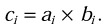This instruction is executed by a pipelined unit able to multiply scalars. The multiplication of two scalars is partitioned into m stages, and the unit can simultaneously perform different stages for different pairs of scalar elements of the vector operands. The execution of vector instruction c = a x b, where a, b, and c are n-element vector registers, by the pipelined unit can be summarized as follows:

• At the first step, the unit performs stage 1 of the multiplication of elements a1 and b1: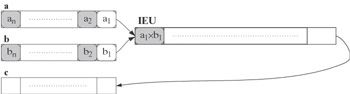• At the ith step (i = 2,…, m - 1), the unit performs in parallel stage 1 of the multiplication of elements ai and bi, stage 2 of the multiplication of elements ai-1 and bi-1, etc., and stage i of the multiplication of elements a1 and b1,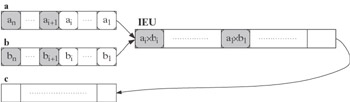• At the mth step, the unit performs in parallel stage 1 of the multiplication of elements am and bm, stage 2 of the multiplication of elements am-1 and bm-1, etc., as well as the final stage m of the multiplication of elements a1 and b1, resulting in a1 X b1 put into element c1 of vector register c: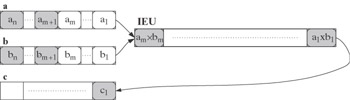• At the (m + j)-th step (j = 1,…, n - m), the unit performs in parallel stage 1 of the multiplication of elements am+j and bm+j, stage 2 of the multiplication of elements am+j-1 and bm+j-1, etc., as well as the final stage m of the multiplication of elements aj+1 and bj+1, resulting in aj+1 X bj+1 put into element cj+1of vector register c: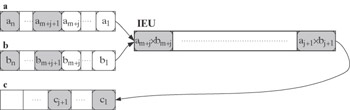• At the (n + k - 1)-th step (k = 2,…, m - 1), the unit performs in parallel stage k of the multiplication of elements an and bn, stage k + 1 of the multiplication of elements an-1 and bn-1, etc., as well as the final stage m of the multiplication of elements an-m+k and bn-m+k, resulting in an-m+k X bn-m+k put into element cn-m+k of vector register c: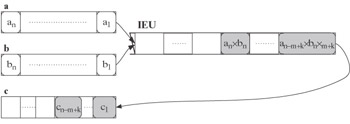• At the (n + m - 1)-th step, the unit only performs the final stage m of the multiplication of elements an and bn, resulting in an X bn put into element cn of vector register c:In total, it takes n + m - 1 steps to execute this instruction. The pipeline of the unit is fully loaded only from the mth to the nth step of the execution. Serial execution of n scalar multiplications with the same unit would take n X m steps. Thus the speedup provided by this vector instruction isIf n is large enough, the speedup is approximately equal to the length of the unit’s pipeline, S m.

Vector architectures are able to speed up such applications, a significant part of whose computations falls into the basic elementwise operations on arrays. Vector architecture includes the serial scalar architecture as a particular case (n = 1, m = 1).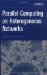Parallel Computing on Heterogeneous Networks (Wiley Series on Parallel and Distributed Computing)
ISBN: B000W7ZQBO
EAN: N/A
Year: 2005
Pages: 95